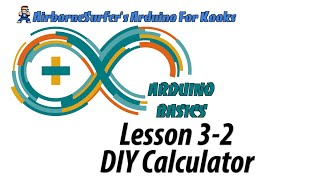# Arduino Basics Lesson 3-3: DIY Calculator

For the “Arduino For Kooks” course, I recommend you get the Arduino Starter Kit available here.

As we’ve learned throughout this series, a microcontroller like the one on the Arduino is basically a rudimentary computer that can execute instruction sets very quickly because it doesn’t have to load all those pesky applications and operating systems. What better capstone for this basic course than to combine everything that we’ve learned so far and build our own rudimentary mathematical computation device: a simple calculator!

For this calculator, we’re going to use a basic 4×4 matrix keypad and attach it to the Arduino in such a way that we only need to use 8 pins to cover the functions of 16 individual switches!

### The Circuit:Connect the character LCD and potentiometer as we learned in the Liquid Crystal Ball project, using the Arduino pins shown in the diagram. You can use a breadboard to create busses for +5V and GND.

Connect the pins of the keypad to pins D0-D8 on the Arduino as shown.### The Sketch:

``` #include //Header file for LCD from https://www.arduino.cc/en/Reference/LiquidCrystal #include //Header file for Keypad from https://github.com/Chris--A/Keypad `````` const byte ROWS = 4; // Four rows const byte COLS = 4; // Three columns `````` // Define the Keymap char keys[ROWS][COLS] = { `````` {'7','8','9','D'}, {'4','5','6','C'}, {'1','2','3','B'}, {'','0','#','A'} }; `````` byte rowPins[ROWS] = { 0, 1, 2, 3 };// Connect keypad ROW0, ROW1, ROW2 and ROW3 to these Arduino pins. byte colPins[COLS] = { 4, 5, 6, 7 }; // Connect keypad COL0, COL1 and COL2 to these Arduino pins. `````` Keypad kpd = Keypad( makeKeymap(keys), rowPins, colPins, ROWS, COLS ); // Create the Keypad `````` const int rs = 8, en = 9, d4 = 10, d5 = 11, d6 = 12, d7 = 13; //Pins to which LCD is connected LiquidCrystal lcd(rs, en, d4, d5, d6, d7); `````` long Num1,Num2,Number; char key,action; boolean result = false; `````` void setup() { lcd.begin(16, 2); //We are using a 16``````2 LCD display lcd.print("DIY Calculator"); //Display a intro message lcd.setCursor(0, 1); // set the cursor to column 0, line 1 lcd.print("-CircuitDigest"); //Display a intro message `````` delay(2000); //Wait for display to show info lcd.clear(); //Then clean it } `````` void loop() { `````` key = kpd.getKey(); //storing pressed key value in a char `````` if (key!=NO_KEY) DetectButtons(); `````` if (result==true) CalculateResult(); `````` DisplayResult(); } `````` void DetectButtons() { lcd.clear(); //Then clean it if (key=='') //If cancel Button is pressed {Serial.println ("Button Cancel"); Number=Num1=Num2=0; result=false;} `````` if (key == '1') //If Button 1 is pressed {Serial.println ("Button 1"); if (Number==0) Number=1; else Number = (Number``````10) + 1; //Pressed twice } `````` if (key == '4') //If Button 4 is pressed {Serial.println ("Button 4"); if (Number==0) Number=4; else Number = (Number10) + 4; //Pressed twice } `````` if (key == '7') //If Button 7 is pressed {Serial.println ("Button 7"); if (Number==0) Number=7; else Number = (Number``````10) + 7; //Pressed twice } `````` if (key == '0') {Serial.println ("Button 0"); //Button 0 is Pressed if (Number==0) Number=0; else Number = (Number10) + 0; //Pressed twice } `````` if (key == '2') //Button 2 is Pressed {Serial.println ("Button 2"); if (Number==0) Number=2; else Number = (Number``````10) + 2; //Pressed twice } `````` if (key == '5') {Serial.println ("Button 5"); if (Number==0) Number=5; else Number = (Number10) + 5; //Pressed twice } `````` if (key == '8') {Serial.println ("Button 8"); if (Number==0) Number=8; else Number = (Number``````10) + 8; //Pressed twice } `````` if (key == '#') {Serial.println ("Button Equal"); Num2=Number; result = true; } `````` if (key == '3') {Serial.println ("Button 3"); if (Number==0) Number=3; else Number = (Number10) + 3; //Pressed twice } `````` if (key == '6') {Serial.println ("Button 6"); if (Number==0) Number=6; else Number = (Number``````10) + 6; //Pressed twice } `````` if (key == '9') {Serial.println ("Button 9"); if (Number==0) Number=9; else Number = (Number10) + 9; //Pressed twice } `````` if (key == 'A' || key == 'B' || key == 'C' || key == 'D') //Detecting Buttons on Column 4 { Num1 = Number; Number =0; if (key == 'A') {Serial.println ("Addition"); action = '+';} if (key == 'B') {Serial.println ("Subtraction"); action = '-'; } if (key == 'C') {Serial.println ("Multiplication"); action = '``````';} if (key == 'D') {Serial.println ("Devesion"); action = '/';} `````` delay(100); } } `````` void CalculateResult() { if (action=='+') Number = Num1+Num2; `````` if (action=='-') Number = Num1-Num2; `````` if (action=='') Number = Num1Num2; `````` if (action=='/') Number = Num1/Num2; } `````` void DisplayResult() { lcd.setCursor(0, 0); // set the cursor to column 0, line 1 lcd.print(Num1); lcd.print(action); lcd.print(Num2); `````` if (result==true) {lcd.print(" ="); lcd.print(Number);} //Display the result `````` lcd.setCursor(0, 1); // set the cursor to column 0, line 1 lcd.print(Number); //Display the result }```

### Congratulations!

If you have completed all 9 lessons in this course, then you have completed the Arduino for Kooks basic-level course! You have learned most of the wiring and programming rudiments necessary to go on and build your very own projects with the Arduino platform!

As a reward for your efforts, I have designed a “merit badge” that you can attach to your EDC, patch wall, or a sweet hacker jacket. You’ve worked hard to achieve it, so show off your new skill set!